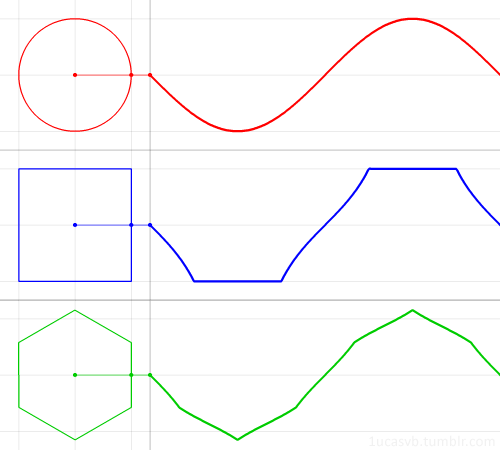# Trigonometría y ondasThe familiar trigonometric functions can be geometrically derived from a circle.

But what if, instead of the circle, we used a regular polygon?

In this animation, we see what the “polygonal sine» looks like for the square and the hexagon. The polygon is such that the inscribed circle has radius 1.

We’ll keep using the angle from the x-axis as the function’s input, instead of the distance along the shape’s boundary. (These are only the same value in the case of a unit circle!) This is why the square does not trace a straight diagonal line, as you might expect, but a segment of the tangent function. In other words, the speed of the dot around the polygon is not constant anymore, but the angle the dot makes changes at a constant rate.

Since these polygons are not perfectly symmetrical like the circle, the function will depend on the orientation of the polygon.

Visto en: FreshPhotons